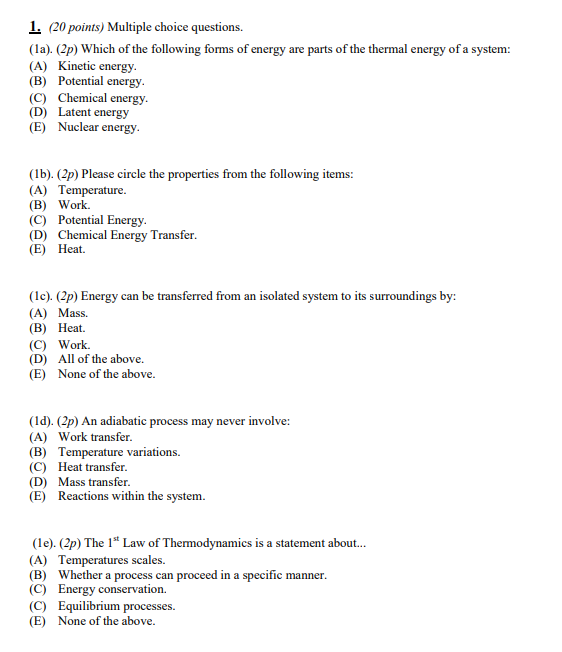Home / Answered Questions / Other / 1-20-points-multiple-choice-questions-1a-2p-which-of-the-following-forms-of-energy-are-parts-of-the--aw749

# (Solved): 1. (20 Points) Multiple Choice Questions. (1a). (2p) Which Of The Following Forms Of Energy Are Part...1. (20 points) Multiple choice questions. (1a). (2p) Which of the following forms of energy are parts of the thermal energy of a system: (A) Kinetic energy. (B) Potential energy. (C) Chemical energy. (D) Latent energy (E) Nuclear energy (1b). (2p) Please circle the properties from the following items: (A) Temperature. (B) Work (C) Potential Energy. (D) Chemical Energy Transfer. (E) Heat. (1c). (2p) Energy can be transferred from an isolated system to its surroundings by: (A) Mass. (B) Heat. (C) Work (D) All of the above. (E) None of the above. (id). (2p) An adiabatic process may never involve: (A) Work transfer. (B) Temperature variations. (C) Heat transfer. (D) Mass transfer. (E) Reactions within the system. (le). (2p) The 1" Law of Thermodynamics is a statement about.. (A) Temperatures scales. (B) Whether a process can proceed in a specific manner. (C) Energy conservation. (C) Equilibrium processes. (E) None of the above.

We have an Answer from Expert